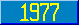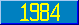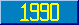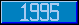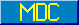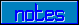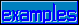## Arithmetic Operators

### 7.2.1.2 Arithmetic binary operators

The binary operators + - * / \ # ** are called the arithmetic binary operators. They operate on the numeric interpretations of their operands, and they produce numeric (in one case, integer) results.

 + produces the algebraic sum. - produces the algebraic difference. * produces the algebraic product. / produces the algebraic quotient. Note that the sign of the quotient is negative if and only if one operand is positive and one operand is negative. Division by zero causes an error condition with ecode="M9". \ produces the integer interpretation of the result of the algebraic quotient. # produces the value of the left operand modulo the right argument. It is defined only for nonzero values of its right operand, as follows. A # B = A (B * floor(A/B)) where floor (x) = the largest integer '> x. ** produces the exponentiated value of the left operand, raised to the power of the right operand. Results producing complex numbers (eg, even numbered roots of negative numbers) are not defined.